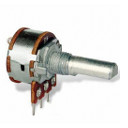# Ashley Sheridan​.co.uk

## Getting Discrete Values from a Potentiometer

Posted onTags:

If you've ever worked with potentiometers in your Arduino sketches, then you might have tried to use them to get discrete values, and if you had, you will have noticed that when the potentiometer is right on the cusp between two of your set value areas that the input appears to fluctuate wildly because it can't settle on one value, and there's a little noise on the line. This problem is compounded if you're absolutely depending on that value being stable.

There are special kinds of potentiometer that you can use in your circuits instead, but they use more inputs. It can be done with a little bit of code though, using a technique that works with something called the hysteresis window.

The basic concept is simple. Consider you want to be able to select between 3 things: a, b, & c, and that you're on option a already, and your potentiometer input will supply values between 0 and 1023:

OptionValue Range
a0 to 341
b341 to 682
c682 to 1023

To move up (that is, from a to b, and from b to c) you would just check to see if the input is greater than the low range point. To move back down the options, you would check to see if the input is less than the high range point with a threshold. It's this threshold that's the key, and it should be something suitable low, like 3, depending on how much noise is actually on the wires.

So, for example, to move from a to b the input needs to be above 341. Now, you're on option b and you want to go back to a. This time, the input needs to fall below 338 (that's 341 - 3, the threshold). The idea is that noise on the wires will only affect the values slightly in one direction and then stop, and won't fall back down because the threshold means that it needs to fluctuate even more.

I wrote the following code to take the input from a potentiometer and a number of discrete options you wish to have this used for (e.g. what options this maps back to) and it will return the option that this input maps to:

``` int options = 12; int selected_option = 1; int threshold = 3; // other script bits void loop() { potVal = analogRead(input); selected_option = calculate_option(selected_option, potVal); Serial.println(selected_option); delay(10); } int calculate_option(int current_option, int input_value) { if(input_value >= option_value(current_option + 1)) { current_option++; } else if(input_value <= (option_value(current_option) - threshold) ) { current_option--; } return current_option; } int option_value(int current_option) { return (1024 / (options - 1) ) * (current_option - 1); } ```

The key functions I wrote here are `calculate_option()` and `option_value()`. The last function just calculates the low value for a range, given the number of discrete options specified by the global `options` variable (which is set to 12 in this example on line 1). The reason for only calculating the low value is that the high one is already shared by the next option as its own low, so generating both values would be redundant.

The `calculate_option()` function accepts the `current_option` (which is set to 1 in `selected_option` at the start of the script), and the input value direct from the potentiometer. It then checks to see if this is greater than the low value for the next option (i.e. is the input enough to make it go up to the next option). If it's not, it checks to see if it's lower than the current options low value minus the threshold (i.e. the input has fallen low enough to go down to the next option).

All in all, this is a fairly simple example of the hysteresis window, and isn't a perfect example. For instance, if the potentiometer is set to some high value when the Arduino is first turned on, the code will cycle through all options beginning at 1 until it reaches the one that matches the input. You can test this yourself by stopping auto-scroll on the serial monitor and looking at the first few lines of output. Hopefully though, this will help if you run into a similar situation in the future and need discrete values from your potentiometers.